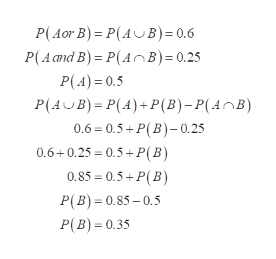# Find the indicated probability. If​ P(A or ​B)equals=0.6, P(A)equals=0.5​, and​ P(A and ​B)equals=0.25​, find​ P(B).

Question
1 views
Find the indicated probability.

If​ P(A or ​B)equals=0.6, P(A)equals=0.5​, and​ P(A and ​B)equals=0.25​, find​ P(B).
check_circle

Step 1

Given that

P (A or B) = 0.6

P (A) = 0.5

P (A and B) = 0.25

Find P (B)

Step 2help_outlineImage TranscriptioncloseP(Aor B) = P(AUB)= 0.6 P(A cmd B) = P(A OB)= 0.25 P(4) = 0.5 P(AUB) = P(4)+P(B)-P(A^B) 0.6 = 0.5+ P(B)-0.25 0.6+0.25 = 0.5+ P(B) 0.85 = 0.5+ P(B) P(B) = 0.85 – 0.5 P(B) = 0.35 fullscreen

### Want to see the full answer?

See Solution

#### Want to see this answer and more?

Solutions are written by subject experts who are available 24/7. Questions are typically answered within 1 hour.*

See Solution
*Response times may vary by subject and question.
Tagged in

### Basic Probability